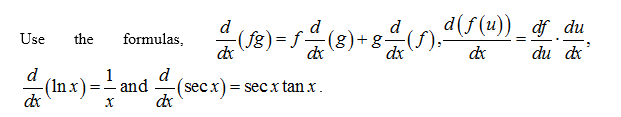# find the derivative of f(x)=sec(x^4)ln(9x-8)

Question

find the derivative of f(x)=sec(x^4)ln(9x-8)

check_circle

Step 1Step 2

Obtain the derivative of the function as follows.

Step 3

On further simpli...

### Want to see the full answer?

See Solution

#### Want to see this answer and more?

Solutions are written by subject experts who are available 24/7. Questions are typically answered within 1 hour.*

See Solution
*Response times may vary by subject and question.
Tagged in

### Derivative